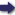## Bīrūnī, Muḥammad ibn Aḥmad, Alberuni's India (v. 2)

(London :  Kegan Paul, Trench, Trübner & Co.,  1910.)

 Tools

## Search this bookPrev Page 55 Next``` CHAPTER LIII. 55 which in the method of ahargana deviate to some other process. Unfortunately that which we possess of the book is badly translated. What we are able to quote from it is the following :—• He subtracts 821 from the years of the Sakakala. The remainder is the basis. This would be the year 132 for our gauge-year. He writes down this number in three different places. He multiplies the first num¬ ber by 132 degrees. The product gives the number 17,424 for our gauge-date. He multiplies the second number by 46 minutes, and gets the product 6072. He multiplies the third number by 34, and gets the product 4488. He divides it by 50, and the quotient represents minutes, seconds, &c., viz. 89' ^6". Then he adds to the sum of degrees in the upper place 112, changing the seconds to minutes, the minutes to degrees, the degrees to circles. Thus he gets 48 circles 358° 41' 46''. This is the mean place of the moon when the sun enters Aries. • Further, he divides the degrees of the mean place of the moon by 12. The quotient represents days. The remainder of the division he multiplies by 60, and adds thereto the minutes of the mean place of the moon. He divides the sum by 12, and the cjuotient represents Page 230. ghatis and minor portions of time. Thus we get 27° 23' 29", i.e. ctdhimdsa days. No doubt this number represents the past portion of the adhimdsa month, which is at present in the course of formation. The author, in regard to the manner in which the measure of the ctdhimdsa month is found, makes the following remark:— He divides the lunar number which we have men¬ tioned, viz. 132° 46' 34", by 12. Thereby he gets as the portio anni 11° 3' 52' 50'", and as the portio mensis 0° 55' 19'' 24"' lO'''. By means of the latter portio he computes the duration of the time in which 30 days sum up as 2 years, 8 months, 16 days, 4 ghati, 45 ```Prev Page 55 Next